### Tension-only and compression-only materials.

These are material models which can be used to simulate textile behavior (tension-only) and concrete (compression-only). In essence, a one-dimensional Hooke-type relationship is established between the principal stresses and principal strains, thereby suppressing the compressive stress range (tension-only materials) or tensile stress range (compression-only materials).

The Cauchy-Green tensor can be written as a function of its eigenvalues and eigenvectors as follows: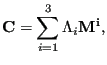(309)

where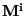are the structural tensors satisfying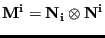,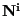being the principal directions . From this, the second Piola-Kirchhoff stress tensor can be defined by: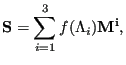(310)

where, for tension-only materials,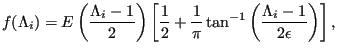(311)

where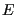is an elastic modulus, the term within the first parentheses is a Lagrange principal strain and the term within the square brackets is a correction term suppressing the negative stresses (pressure). It is a function tending to zero for negative strains (-0.5 being the smallest possible Lagrange strain), to one for large positive strains and switches between both in a region surrounding zero strain. The extent of this region is controlled by the parameter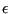: the smaller its value, the smaller the transition region (the sharper the switch). It is a monotonically increasing function of the strain, thus guaranteeing convergence. The correction term is in fact identical to the term used to cut off tensile stresses for penalty contact in Equation(203) and Figure (130). Replacing “overclosure” and “pressure” by “principal strain” and “principal stress” in that figure yields the function f. Although compressive stresses are suppressed, they are not zero altogether. The maximum allowed compressive stress (in absolute value) amounts to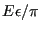. Instead of chosingandthe user definesand the maximum allowed compressive stress, from whichis determined.

The material definition consists of a *MATERIAL card defining the name of the material. This name HAS TO START WITH ”TENSION_ONLY” but can be up to 80 characters long. Thus, the last 68 characters can be freely chosen by the user. Within the material definition a *USER MATERIAL card has to be used satisfying:

First line:

• *USER MATERIAL
• Enter the CONSTANTS parameter and its value. The value of this parameter is 2.

Following line:

•.
• absolute value of the maximum allowed pressure.
• Temperature.
Repeat this line if needed to define complete temperature dependence.

For a compression-only materials the name of the material has to start with ”COMPRESSION_ONLY” (maximum 64 characters left to be chosen by the user) and the second constant is the maximum allowed tension. Examples are leifer2 and concretebeam in the test example suite.